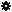﻿ Object Model: PolygonFace.NeighborPolygons

PolygonFace.NeighborPolygons

## PolygonFace.NeighborPolygonsv3.0

### Description

Returns a collection of PolygonFace neighbors within a given distance. If the distance is 1 all PolygonFace objects that share an Edge with the current PolygonFace are returned in counter-clockwise order.

### C# Syntax

 ```PolygonFaceCollection PolygonFace.NeighborPolygons( Int32 in_lDistance ); ```

### Scripting Syntax

 ```oReturn = PolygonFace.NeighborPolygons( [Distance] ); ```

### Parameters

Parameter Type Description
Distance Integer Distance is an integer value representing the degree of neighborhood (eg: degree=2 for a polygon means its adjacent polygons plus the adjacent polygons of the adjacent polygons)

Default Value: 1

### Examples

#### VBScript Example

 ```set oCube = ActiveSceneRoot.AddGeometry( "Cube", "MeshSurface" ) set oGeometry = oCube.ActivePrimitive.Geometry for each Polygon in oGeometry.Polygons set oNeighbors = Polygon.NeighborPolygons( 1 ) str = "PolygonFaces neighbors of face( " & Polygon.index & ") are :" for each neighborPolygon in oNeighbors str = str & " " & neighborPolygon.index next logmessage str next ```